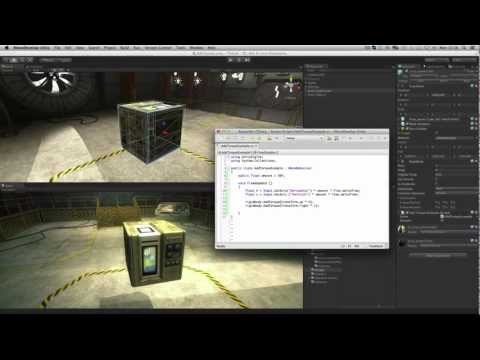# Adding Physics Torque

#### -

How to use Add Torque to rotate physics (rigidbody) objects.## Adding Physics Torque

Anfänger Physics

Abschriften

### Code snippet

``````using UnityEngine;
using System.Collections;

public class AddTorqueExample : MonoBehaviour
{
public float amount = 50f;

void FixedUpdate ()
{
float h = Input.GetAxis("Horizontal") * amount * Time.deltaTime;
float v = Input.GetAxis("Vertical") * amount * Time.deltaTime;

}
}``````
``````#pragma strict

public var amount : float = 50f;

function FixedUpdate ()
{
var h : float = Input.GetAxis("Horizontal") * amount * Time.deltaTime;
var v : float = Input.GetAxis("Vertical") * amount * Time.deltaTime;

}``````
``````import UnityEngine
import System.Collections

public amount as single = 50.0F

private def FixedUpdate():
h as single = ((Input.GetAxis('Horizontal') * amount) * Time.deltaTime)
v as single = ((Input.GetAxis('Vertical') * amount) * Time.deltaTime)